GET VISIBLE! Advertise Here. Find Out More

 #slider468x60 { position: relative; width: 750px; height: 90px; } #slider468x60 > div { position: absolute; }A Full Body Cellular Oxygen DetoxRapid cellular absorption - Amazing picometer ionic 60,000ppm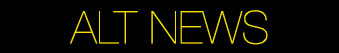Dr. Randy Mills
Electrolysis Explained

I put this together to help you see the electrical energy flows and the sub atomic energy flows doing the work.

The first measurable energy is the amount of electrical current doing work as an electrical force acting on atoms. The energy of charged electrons in the oxidation and reduction of water electrolysis coming from a battery are shown here. 237 kJ of energy are required for one mole of H2O(l) to be transformed. That is a measureable fact

 Cathode reduction: 2 H2O(l) + 2e− → H2(g) + 2 OH−(aq)

Oxidation at anode: 2 H2O(l) → O2(g) + 4 H+(aq) + 4e

The balance total equation can be formed by adding the two equations. To do this the reduction equation is multiplied by 2:

 Cathode reduction: 2x (2 H2O(l) + 2e− → H2(g) + 2 OH−(aq))

Oxidation at anode: 2 H2O(l) → O2(g) + 4 H+(aq) + 4e

And then the equations are combined

4 H2O(l) + 4e + 2 H2O(l) → 2H2(g) + 4 OH(aq) + O2(g) + 4 H+(aq) + 4e

This factors down to this by removing the 4e-

4 H2O(l) + 2 H2O(l) → 2H2(g) + 4 OH(aq) + O2(g) + 4 H+(aq)

Then to this by combining the aqueous ions 4 OH(aq) + 4 H+(aq) → 4H2O(l)

6 H2O(l) → 2H2(g) + O2(g) + 4H2O(l)

Subtracting 4H2O(l) from each side gives

2 H2O(l) → 2H2(g) + O2(g)

There were no electrons placed into the atoms of the Hydrogen and Oxygen while this work was done, because the 4 electrons continued to pass to the other side of the battery which is where the electrons changed hands going from the cathode to the anode.

UC Berkley already proved electrolysis of water is 100% efficient. This is been verified to be true. Otherwise the leaves of plants would heat up and catch fire on a sunny day from the heat caused by the conduction of electromagnetic radiation absorbed by photosystem I & photosystem II.

Now comes the first problem with the above explanation from the thermodynamics observation of burning hydrogen and oxygen to yield exactly one mole of water. That process yields 286 kJ of energy which is measured using a calorimeter. How is that possible? That is 49 kJ ( 286kJ - 237 kJ = 49 kJ ) more energy than was measured electrically put into the electrolysis reaction?

To understand this you must first look at the phase diagram from Thermodynamics that says that 49 kJ of energy is required to move one mole of water from a liquid to a gas.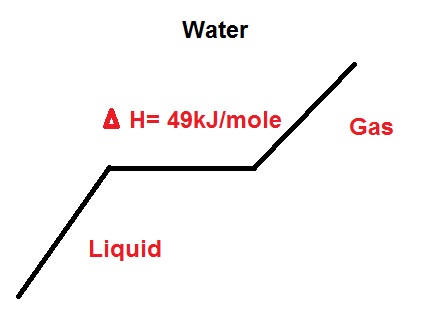So we know where the extra 49 kJ is supposed to come from in combustion. This can also be measured using thermometers and calorimeters by naturally heating water to a gas and looking at the inputs and outputs.

Now back to the Hydrolysis reaction whereby 237 kJ of electrons was put into the process, but you got Hydrogen and Oxygen gas that could be combusted to release 286 kJ of water to form water.

The 49 kJ came from when the electrical current exerted atomic strain and force upon the water molecules at the electrodes of the system. This strain affects the atomic electrical orbits of the Hydrogen and Oxygen and destabilizes them. In fact the electrical force of the electrons works upon the gravitational, weak, strong, and nuclear forces of both the Hydrogen and Oxygen atoms while destabilizing the water molecule.

This is how Stan Meyers illustrated this behavior in his patents: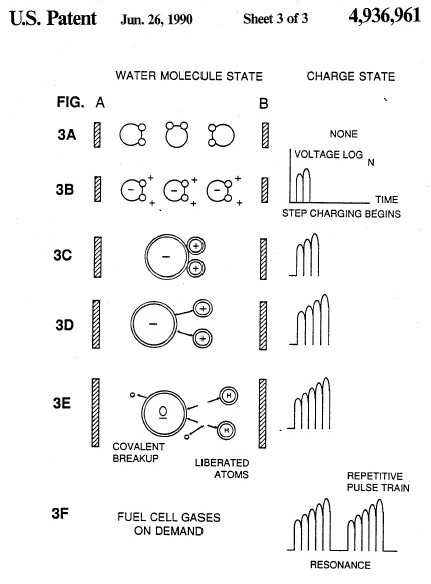Here is a zoom in on the orbitals of the water molecule: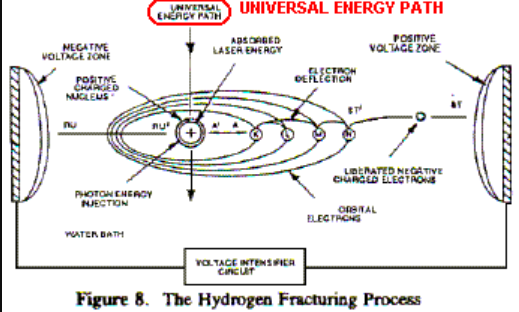And here is another one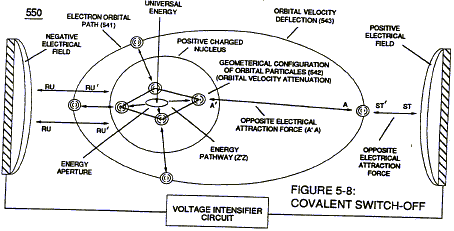To fully understand what is occurring you must mentally visualize the behavior of the electrons in the orbitals. Here is Hydrogen portrayed in multiple orbital states: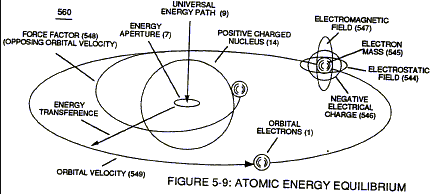Here is Oxygen using a Bohr model to shoe the electron orbitals: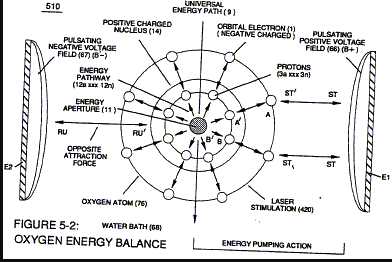What is happening to these electron orbitals during the fracturing of water by electrical energy forces acting upon them is then shown by further zooming in to a picture that depicts the interaction of the subatomic particles from a "Very High level" Universal Energy Diagram diagram (below) showing you the universal pathway that energy comes into the Hydrogen atom (or any atom) to stabilize it after this strain on the electron orbitals occurs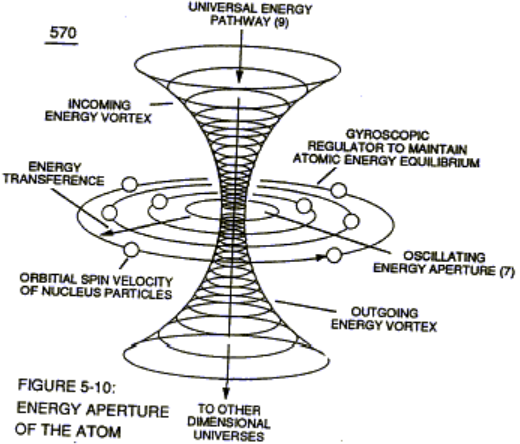I said this was a high level diagram. At the time we did not have better diagrams to help explain how the energy came into the Hydrogen atom to stabilize it. In fact CERN and several other facilities had not yet been built to look deeper into the subatomic particle function. Here is my drawing to show the energy transfer into the Gluons through the Universal Pathway to stabilize the electrons of the Hydrogen atom from a water molecule.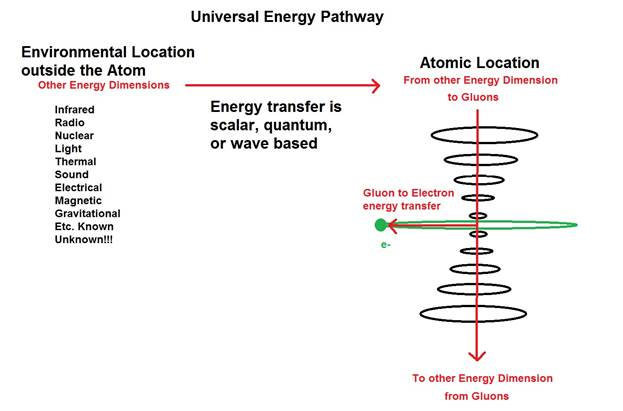And now you see how the orbital distance and speed of the electron can be maintained in the Hydrogen atom during its strain and stress during the fracturing of a water molecule during water electrolysis using electrons so that the Hydrogen atom does not implode or explode to cause a thermonuclear reaction. These states of the electrons in the Hydrogen atom have now been observed as Hydrino states and there are 138 of them.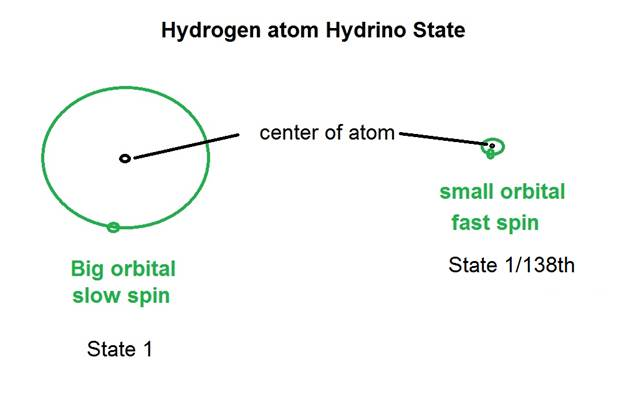This picture illustrates the intermediates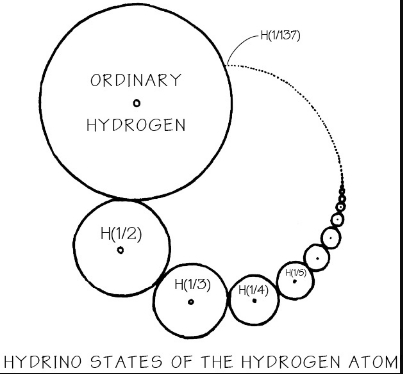Back to the water hydrolysis chemical equation that is unbalanced!

2 H2O(l) → 2H2(g) + O2(g)

Wait, we used electrical power from a battery, so

4e+ 2 H2O(l) → 2H2(g) + O2(g) + 4e

But we know from thermodynamics and measurements that more energy went into this, where is it in the equation?

Here it is:

Energy from surroundings to Gluons + 4e+ 2 H2O(l) → 2H2(g) + O2(g) + 4e + Energy lost from Gluons to environment

And that is why you got a clean phase change from liquid to gas per the laws of thermodynamics. That energy input happens to have been measured and is exactly 49 kJ/mole. There was no heat observed because the energy went to the electron spin of the atom.

There likely was energy lost during the stress and strain of the fracturing in the Universal pathway. So it is included in the equation. The important thing to realize is that the total net input stabilizing the system was 49 kJ/mole of water.

Everything here has been measure in labs doing many experiments over hundreds of years. The other 20.7% energy of hydrogen and oxygen that did not come from electrical input came from input from the surroundings into the Gluon sub atomic particles during the energy fracturing process. Therefore the energy input is conserved as well as the energy output per the Law of Conservation of Energy.

This is also tied to the special relativity equation E = mc2 because energy is used to conserve the mass of the atoms and molecules. Otherwise, the loss of energy in the electron orbitals during the water fracturing would have resulted in a mass loss, but that is not possible either by the reaction of the visually (with eyes and a scale) observed:

2 H2O(l) → 2H2(g) + O2(g)